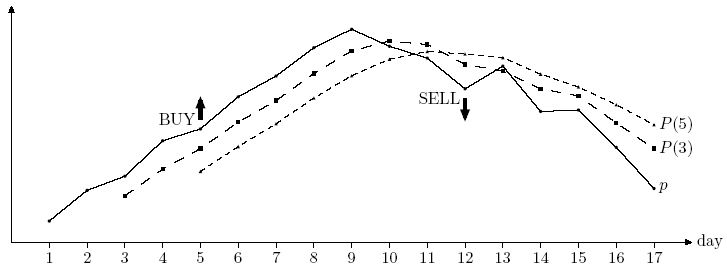Online JudgeProblem SetAuthorsOnline ContestsUser
Web Board
F.A.Qs
Statistical Charts
Problems
Submit Problem
Online Status
Prob.ID:
Register
Authors ranklist
Current Contest
Past Contests
Scheduled Contests
Award Contest
Register
Language:
 Time Limit: 1000MS Memory Limit: 65536K Total Submissions: 2672 Accepted: 1144 Special Judge

Description

Frank is a professional stock trader for Advanced Commercial Markets Limited (ACM Ltd). He likes "easy trading" -- using a straightforward strategy to decide when to buy stock and when to sell it. Frank has a database of historical stock prices for each day. He uses two integer numbers m and n (1 <= m < n <= 100) as parameters of his trading strategy. Every day he computes two numbers: P(m) -- an average stock price for the previous m days, and P(n) — an average stock price for the previous n days. P(m) > P(n) is an indicator of the upward trend (traders call it bullish trend), and P(m) < P(n) is an indicator of the downward trend (traders call it bearish trend). In practice the values for P(m) and P(n) are never equal.
When a trend reverses from bearish to bullish it is a signal for Frank to buy stock. When a trend reverses from bullish to bearish it is a signal to sell.Frank has different values for m and n in mind and he wants to backtest them using historical prices. He takes a set of k (n < k <= 10 000) historical prices pi (0 < pi < 100 for 1 <= i <= k). For each i (n <= i <= k) he computes Pi(m) and Pi(n) — an arithmetic average of pi-m+1 . . . pi and pi-n+1 . . . pi respectively. Backtesting generates trading signals according to the following rules.
• If Pi(m) > Pi(n) there is a bullish trend for day i and a "BUY ON DAY i" signal is generated if i = n or there was a bearish trend on day i - 1.
• If Pi(m) < Pi(n) there is a bearish tread for day i and a "SELL ON DAY i" signal is generated if i = n or there was a bullish trend on day i - 1.

Your task is to write a program that backtests a specified strategy for Frank -- you shall print a signal for the first tested day (day n) followed by the signals in increasing day numbers.

Input

The first line of the input contains three integer numbers m, n, and k. It is followed by k lines with stock prices for days 1 to k. Each stock price pi is specified with two digits after decimal point. Prices in the input file are such that Pi(m) != Pi(n) for all i (n <= i <= k).

Output

Write to the output a list of signals -- one signal on a line, as described in the problem statement.

Sample Input

```3 5 17
8.45
9.10
9.40
10.15
10.40
11.08
11.52
12.12
12.51
12.15
11.90
11.25
11.73
10.77
10.80
10.01
9.14```

Sample Output

```BUY ON DAY 5
SELL ON DAY 12```

Source

[Submit]   [Go Back]   [Status]   [Discuss]Home PageGo BackTo top